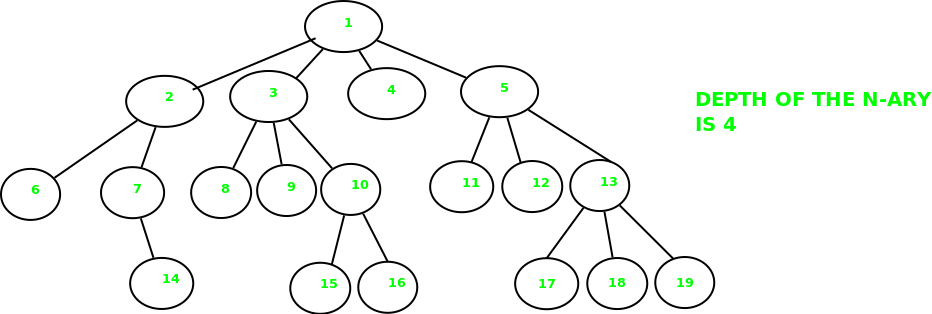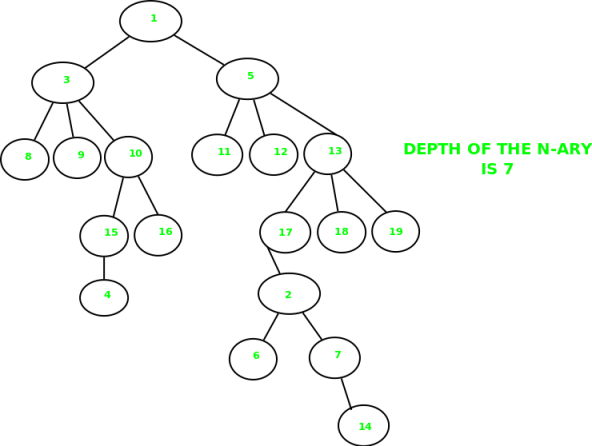# Depth of an N-Ary tree

Given an N-Ary tree, find depth of the tree. An N-Ary tree is a tree in which nodes can have at most N children.

Examples:
Example 1:Example 2:## Recommended: Please try your approach on {IDE} first, before moving on to the solution.

N-Ary tree can be traversed just like a normal tree. We just have to consider all childs of a given node and recursively call that function on every node.

 `// C++ program to find the height of ` `// an N-ary tree ` `#include ` `using` `namespace` `std; ` ` `  `// Structure of a node of an n-ary tree ` `struct` `Node ` `{ ` `   ``char` `key; ` `   ``vector child; ` `}; ` ` `  `// Utility function to create a new tree node ` `Node *newNode(``int` `key) ` `{ ` `   ``Node *temp = ``new` `Node; ` `   ``temp->key = key; ` `   ``return` `temp; ` `} ` ` `  `// Function that will return the depth ` `// of the tree ` `int` `depthOfTree(``struct` `Node *ptr) ` `{ ` `    ``// Base case ` `    ``if` `(!ptr) ` `        ``return` `0; ` ` `  `    ``// Check for all children and find ` `    ``// the maximum depth ` `    ``int` `maxdepth = 0; ` `    ``for` `(vector::iterator it = ptr->child.begin(); ` `                              ``it != ptr->child.end(); it++) ` `        ``maxdepth = max(maxdepth, depthOfTree(*it)); ` ` `  `    ``return` `maxdepth + 1 ; ` `} ` ` `  `// Driver program ` `int` `main() ` `{ ` `   ``/*   Let us create below tree ` `   ``*             A ` `   ``*         / /  \  \ ` `   ``*       B  F   D  E ` `   ``*      / \    |  /|\ ` `   ``*     K  J    G  C H I ` `   ``*      /\            \ ` `   ``*    N   M            L ` `   ``*/` ` `  `   ``Node *root = newNode(``'A'``); ` `   ``(root->child).push_back(newNode(``'B'``)); ` `   ``(root->child).push_back(newNode(``'F'``)); ` `   ``(root->child).push_back(newNode(``'D'``)); ` `   ``(root->child).push_back(newNode(``'E'``)); ` `   ``(root->child->child).push_back(newNode(``'K'``)); ` `   ``(root->child->child).push_back(newNode(``'J'``)); ` `   ``(root->child->child).push_back(newNode(``'G'``)); ` `   ``(root->child->child).push_back(newNode(``'C'``)); ` `   ``(root->child->child).push_back(newNode(``'H'``)); ` `   ``(root->child->child).push_back(newNode(``'I'``)); ` `   ``(root->child->child->child).push_back(newNode(``'N'``)); ` `   ``(root->child->child->child).push_back(newNode(``'M'``)); ` `   ``(root->child->child->child).push_back(newNode(``'L'``)); ` ` `  `   ``cout << depthOfTree(root) << endl; ` ` `  `   ``return` `0; ` `} `

Output:

```4
```

This article is contributed by Shubham Gupta. If you like GeeksforGeeks and would like to contribute, you can also write an article using contribute.geeksforgeeks.org or mail your article to contribute@geeksforgeeks.org. See your article appearing on the GeeksforGeeks main page and help other Geeks.

Please write comments if you find anything incorrect, or you want to share more information about the topic discussed above.

My Personal Notes arrow_drop_up

Article Tags :
Practice Tags :

2

Please write to us at contribute@geeksforgeeks.org to report any issue with the above content.